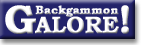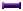Pip CountingModified direct count

 From: Daithi Date: 4 March 2011 Subject: Re: New Pip Counting Methods Forum: 2+2 backgammon forum

```I use the "Direct Count" method.  That's only half a joke.  Here's what I
do.

On the bottom half of my board I start by counting the largest odd numbered
block and multiply the center point of that block by the number of checkers
in the block. For example, if I have a five prime with 2 checkers on each
point and the middle of the block is on the 6 point then I know I have 60
pips (6*10=60). Then I do a direct count of any remaining checkers on the
bottom half of my board adding them to my initial total. So this is just a
modified direct count.

Sometimes I will have two blocks of checkers I need to add together. For
example, at the start of the game I have two odd numbered blocks in the
lower half. I have 3 on the 8 point and 5 on the 6 point. Adding these
together is trivial (3x8) + (5x6) = 54.

Sometimes my block of checkers won't have a uniform number on each point.
This isn't a problem if the center checker of the block contains an extra
checker or two, otherwise I just do a mental adjustment or count that
checker seperately. So if I have a 5 prime with 2 checkers on each point
with the center at the 5 point, but an extra checker on the 6 point then I
have 11 checkers total times 5 is 55 then add 1 to adjust for the checker
on the 6 point. Or do 10 checkers times 5 plus the 6 point checker. Either
way the total is 56.

If I have an even block of checkers, say a 6 prime with 2 checkers on each
point, then I still calculate the block of 5 then just add the other two
checkers. For example, if I have two checkers on all my homeboard points
then I have a five prime from 2 to 6 with 3 as the center point, so this is
30 pips, plus 2 more pips for checkers on the 1 point.

When all the checkers are on the bottom half of the board this technique
makes quick work of the pip count. Even when you have one or two checkers
in the top half of the board it is also trivial to add them to the total.

When I have several checkers in the top half I do the following. I multiply
the number of checkers in the top section by 12 and then do a direct count
as if the checkers were on points 1 through 12. For example, in the
starting position there are 5 checkers on the 13 point and 2 on the 24
point. So this is 7 checkers total (7 x 12 = 84), and then we add 5 for the
checkers on the 13 point (i.e. 1 x 5), and 24 for the checkers on the 24
point (i.e. 2 x 12). This is 113 pips for the checkers in the upper half of
the board.

Oh yeah, if I notice that there are checkers on the 15 or 20 points, I'll
probably just count those directly to make things even easier on myself.

Anyway, that's my modified direct count and it's pretty simple.
```

### Pip Counting

Casting out crossovers  (Mark Denihan, Oct 1996)
Cluster counting  (camelx+, May 2005)
Counting half rolls  (Bob Hoey, Apr 1998)
Half-crossover method  (Douglas Zare, Mar 2002)
Live play versus online  (Stanley E. Richards+, Apr 2006)Live play versus online  (Rich+, Mar 2006)
Mental shift  (Stephen Turner, Oct 1996)
Modified direct count  (Daithi, Mar 2011)
Opposing sums and differences  (Donald Kahn, Apr 1998)
Running pip count  (Rodrigo Andrade+, Apr 1998)
Symmetry method, Grouping men  (Brian Sheppard, Jan 1997)
The 51/21 count  (kruidenbuiltje, Mar 2011)From GammOnLineLong messageRecommended readingRecent addition